Many different question types are available within the Quiz tool.

## Basic Question Types

• Multiple Choice (MC): Ask a question, and present multiple answer options. The student selects the ONE correct answer.
•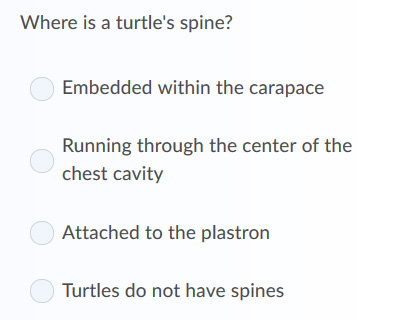• Multiple Select (MS): Ask a question, and present multiple answer options. The student selects the MULTIPLE correct answers.
•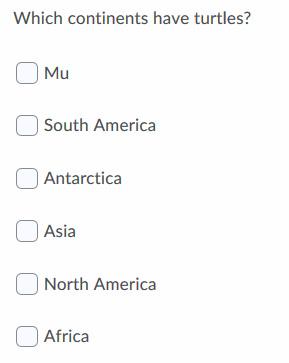• True/False (TF): Make a statement. The student decides if it is True or False.
•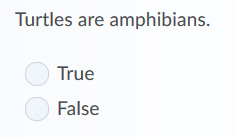• Ordering (ORD): Provide a set of steps or statements. The student chooses the correct order in which they should occur.
•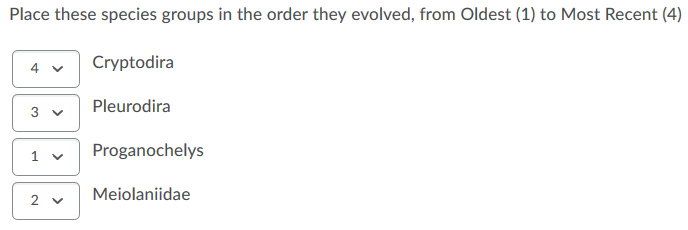• Matching (MAT): Provide a set of Choices and Matches. The student decides which Choice corresponds to each Match.
•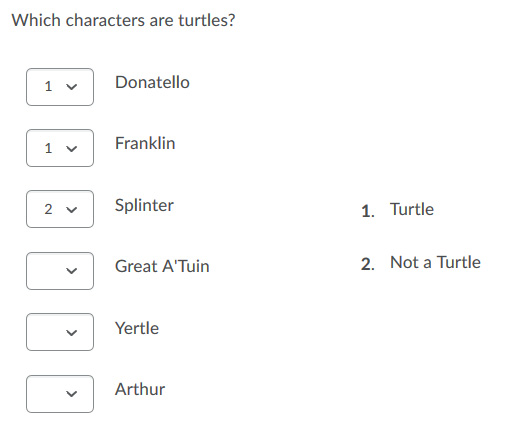## Text-Entry Questions

• Fill In The Blanks: The student must answer a question or complete a sentence using a one-word type-in box. A question can contain multiple blanks. A correct answer must be entered and the system WILL automatically mark the question.
• Short Answer: The student must answer a question using a one-line type-in box. A correct answer must be entered and the system WILL automatically mark the question.
• Written Response: The student must answer a question using a multi-line type-in box. Text formatting can be enabled. The question must be MANUALLY graded by the instructor.
•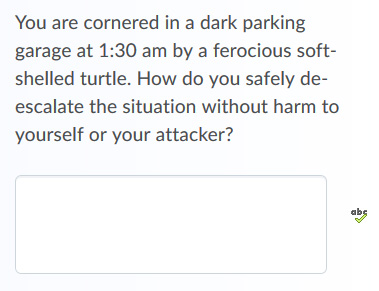In practice, we strongly recommend using Written Response for nearly all questions that require a student to type their answer. Short Answer questions with a multi-word answer will almost never be accurately marked by the system. A student's answer will only be considered correct if it perfectly matches the answer you entered, EXACTLY as you typed it, including all spelling, word order, spacing, and punctuation. Even Fill-In-The-Blanks questions, which typically only involve a one-word answer, will still very frequently penalize a student for variations in spelling, pluralization, and acronym punctuation. As a result, these question types should usually be avoided in favour of Written Response.

The only case where a Fill-In-The-Blanks question might be appropriate is where the answer is a number (and ideally, an integer with no decimals), as students are more likely to be able to enter that answer exactly as the question intended.

## Calculated Questions

• Arithmetic Questions: Establish a set of variables, specifying the range of possible numbers for each one (min, max, and granularity of increments). Teach the system a formula to be calculated using these variables using a special equation editor. Each student will then be provided a different set of numbers for each variable, and the system will evaluate whether they accurately calculated the answer using the specific numbers that student was given to work with.
•• Significant Figures: Exactly like an Arithmetic Question, but the student will be asked to express their answer in Significant Figures format (a x 10^b).